Question

# Consider a circuit with two 10 ohm resistors in series and connected to a 6 V...

Consider a circuit with two 10 ohm resistors in series and connected to a 6 V battery. A certain current flows through it. What would happen to that current if you added a third 10 ohm resistor in series with the other two? Would the current increase, decrease, stay the same, or become zero after you added the third resistor? Give a short justification of your answer.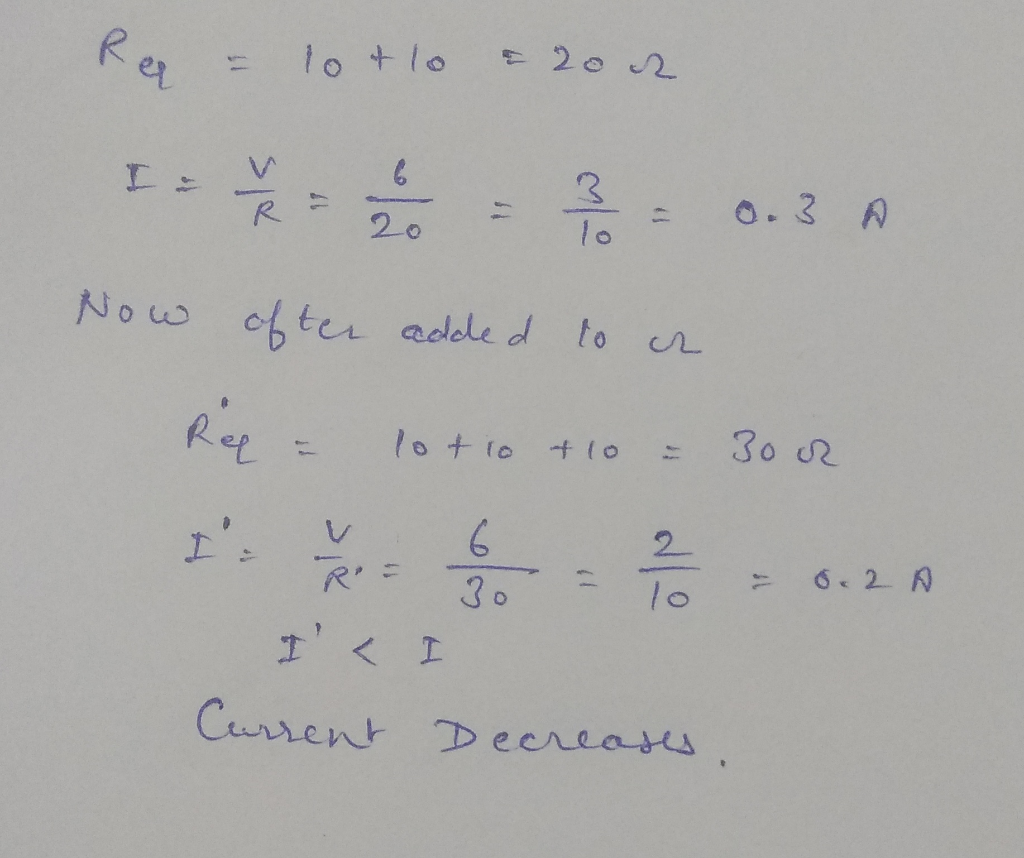#### Earn Coins

Coins can be redeemed for fabulous gifts.

Similar Homework Help Questions
• ### A 120.0 ohm resistor is connected in parallel to a 60.0 ohm resistor. These two resistors...

A 120.0 ohm resistor is connected in parallel to a 60.0 ohm resistor. These two resistors in parallel are then connected in series with a 20.0 ohm resistor. The total combination is connected across a 15.0 V battery. a. Find the total current in the circuit. b. Find the power dissipated in the 120.0 ohm resistor.

• ### Two 5 ohm resistors are connected in series to a 15 V battery. What current flows...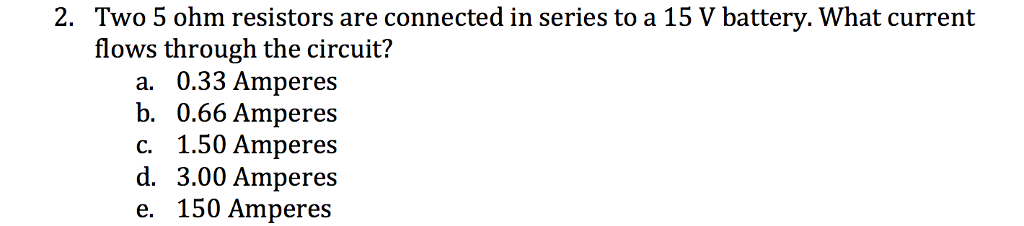Two 5 ohm resistors are connected in series to a 15 V battery. What current flows through the circuit? a. 0.33 Amperes b. 0.66 Amperes c. 1.50 Amperes d. 3.00 Amperes e. 150 Amperes

• ### Two resistors, R1 = 10-ohm and R2 = 20-ohm, are connected in series to each other,...

Two resistors, R1 = 10-ohm and R2 = 20-ohm, are connected in series to each other, and this series combination is connected across a 30-V source of emf. The current through the resistor R2 is:

• ### A 6.0 V battery is connected to two 7.70 kilo-Ohm resistors in series. An ammeter of...

A 6.0 V battery is connected to two 7.70 kilo-Ohm resistors in series. An ammeter of internal resistance 0.570 Ohm measures the current and at the same time a voltmeter with internal resistance 15.2 kilo-Ohm measures the voltage across one of the 7.70 kilo-Ohm resistors in the circuit. What does the ammeter read? What does the voltmeter read?

• ### 6. A three-ohm resistor and a four-ohm resistor are connected in series to a battery. In...

6. A three-ohm resistor and a four-ohm resistor are connected in series to a battery. In a separate circuit, a three-ohm resistor and a four-ohm resistor are connected in parallel to a battery with the same battery voltage as in the first circuit. Which resistor will have the largest amount of current flowing through it? A. The three-ohm resistor in the series circuit. B. The four-ohm resistor in the series circuit. C. The three-ohm resistor and the four-ohm resistor in...

• ### A 24.0 volt battery is connected to three resistors to make a complete circuit. Resistor R1...

A 24.0 volt battery is connected to three resistors to make a complete circuit. Resistor R1 = 15 ohm is in series with two other resistors that are in parallel with each other, R2 = 25.0 ohm and R3 = 15.0 ohm. What is the current that flows through the resister R1? Please explain how the answer is 0.98462 A.

• ### Two resistors, A and B, are connected in parallel across a 7.0 V battery. The current...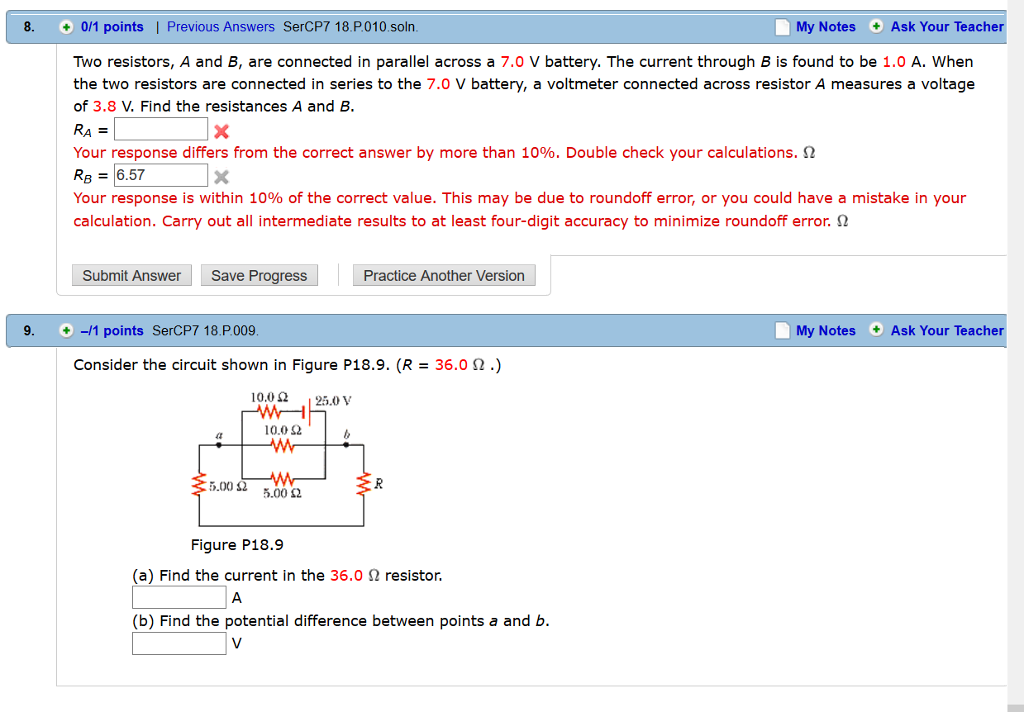Two resistors, A and B, are connected in parallel across a 7.0 V battery. The current through B is found to be 1.0 A. When the two resistors are connected in series to the 7.0 V battery, a voltmeter connected across resistor A measures a voltage of 3.8 V. Find the resistances A and B. R_A = Your response differs from the correct answer by more than 10%. Double check your calculations. ohm R_B = 6.57 Your response is within...

• ### A circuit consists of two resistors in series followed in series by two resistors in parallel....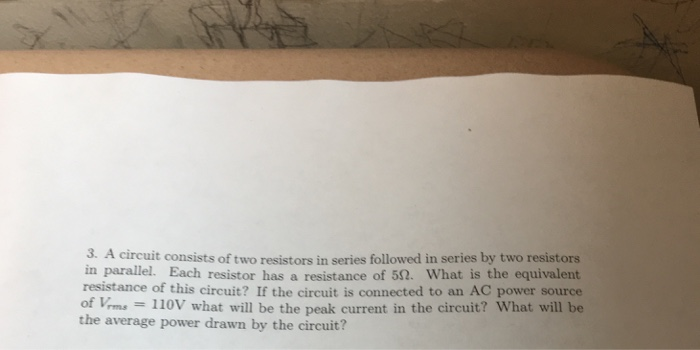A circuit consists of two resistors in series followed in series by two resistors in parallel. Each resistor has a resistance of 5 Ohm. What is the equivalent resistance of this circuit? If the circuit is connected to an AC power source of V_rms = 110V what will be the peak current in the circuit? What will be the average power drawn by the circuit?

• ### Three resistors having resistances of 4.0 Ohm, 6.0 Ohm, and 10.0 Ohm are connected in parallel....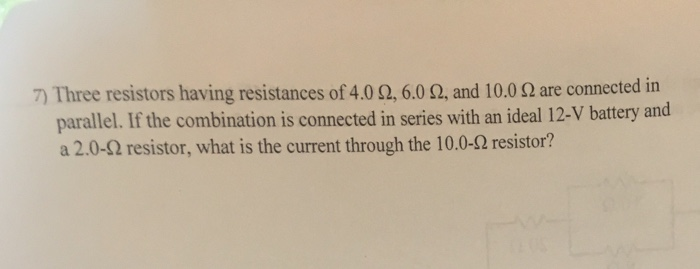Three resistors having resistances of 4.0 Ohm, 6.0 Ohm, and 10.0 Ohm are connected in parallel. If the combination is connected in series with an ideal 12-V battery and a 2.0-Ohm resistor, what is the current through the 10.0-Ohm resistor?

• ### 1. Consider a series circuit consisting of a battery and two resistors. If the current through...

1. Consider a series circuit consisting of a battery and two resistors. If the current through one resistor is 18 A what is the current through the second resistor? 2. connected in parallel across a potential difference of 24.if In a parallel circuit, consisting of two resistors eadh the current put out by the battery is 74 A and the current through one resistor is 36A, what is the current through the other resistor?